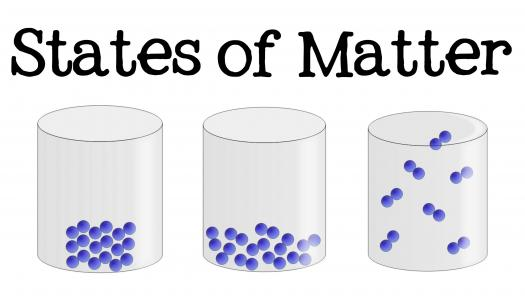# Particular Theory Of Matter

25 Questions | Total Attempts: 84SettingsThe Particle Theory of Matter This theory has five points: 1. All matter is made of extremely tiny particles (much, much smaller than cells!) 2. Each pure substance is made of just one kind of particle 3. Particles attract each other 4. Particles are always in motion 5. The temperature of the particles controls how much they move about.

• 1.
Which of the following is NOT an example of matter?
• A.

Air

• B.

Smoke

• C.

Heat

• D.

Water vapor

• 2.
A golf ball has more mass than a tennis ball because it ____.
• A.

Takes up more space

• B.

Contains more matter

• C.

Contains different kinds of matter

• D.

Has a definite composition

• 3.
An example of an extensive property of matter is ____.
• A.

Mass

• B.

Temperature

• C.

Pressure

• D.

Hardness

• 4.
All of the following are physical properties of matter EXCEPT ___
• A.

Mass

• B.

Color

• C.

Melting point

• D.

Ability to rust

• 5.
Which of the following is NOT a physical property of water?
• A.

It has a boiling point of 100C.

• B.

It is a colorless liquid.

• C.

It is composed of hydrogen and oxygen.

• D.

Sugar dissolves in it.

• 6.
Which of the following is NOT a physical property of water?
• A.

It has a boiling point of 100C.

• B.

It is a colorless liquid.

• C.

It is composed of hydrogen and oxygen.

• D.

Sugar dissolves in it.

• 7.
Which of the following are considered physical properties of a substance?
• A.

Color and odor

• B.

Melting and boiling points

• C.

Malleability and hardness

• D.

All of the above

• E.

• 8.
A vapor is which state of matter?
• A.

Solid

• B.

Liquid

• C.

Gas

• D.

all of the above

• 9.
A substance that forms a vapor is generally in what physical state at room temperature?
• A.

solid

• B.

Liquid or solid

• C.

Gas

• D.

liquid

• 10.
Which state of matter has a definite volume and takes the shape of its container?
• A.

solid

• B.

liquid

• C.

gas

• D.

both b and c

• E.

both a and b

• F.

both a and c

• 11.
Which state of matter takes both the shape and volume of its container?
• A.

Solid

• B.

Liquid

• C.

Gas

• D.

Both b and c

• 12.
Which state of matter is characterized by having an indefinite shape, but a definite volume?
• A.

Gas

• B.

Liquid

• C.

Solid

• D.

None of the above

• 13.
Which state of matter is characterized by having a definite shape and a definite volume?
• A.

Gas

• B.

Liquid

• C.

Solid

• D.

All of the above

• 14.
Which state of matter expands when heated and is easy to compress?
• A.

Gas

• B.

Liquid

• C.

Solid

• D.

All of the above

• 15.
All of the following are physical properties of a substance in the liquid state EXCEPT ____.
• A.

Indefinite volume

• B.

Definite mass

• C.

Not easily compressed

• D.

Indefinite shape

• 16.
Which of the following is a physical change?
• A.

Corrosion

• B.

Explosion

• C.

Evaporation

• D.

Rotting of food

• 17.
Which of the following CANNOT be classified as a substance?
• A.

Table salt

• B.

Air

• C.

Nitrogen

• D.

Gold

• 18.
Which of the following is a heterogeneous mixture?
• A.

Air

• B.

Salt water

• C.

Steel

• D.

Soil

• 19.
Which of the following CANNOT be considered a single phase?
• A.

A pure solid

• B.

A pure liquid

• C.

A homogeneous mixture

• D.

A heterogeneous mixture

• 20.
Which of the following is true about homogeneous mixtures?
• A.

They are known as solutions.

• B.

They consist of two or more phases.

• C.

They have compositions that never vary.

• D.

They are always liquids.

• 21.
Which of the following is a homogeneous mixture?
• A.

Salt water

• B.

Beef stew

• C.

Sand and water

• D.

Soil

• 22.
An example of a homogeneous mixture is ____.
• A.

Water

• B.

Stainless steel

• C.

Noodle soup

• D.

Oxygen

• 23.
Which of the following items is NOT a compound?
• A.

• B.

Baking soda

• C.

Sucrose

• D.

Table salt

• 24.
Which of the following is true about compounds?
• A.

They can be physically separated into their component elements.

• B.

They have compositions that vary.

• C.

They are substances.

• D.

They have properties similar to those of their component elements.

• 25.
Which of the following materials is a substance?
• A.

Air

• B.

Gasoline

• C.

Stainless steel

• D.

Silver

Related TopicsBack to top